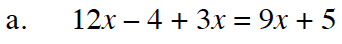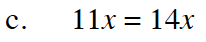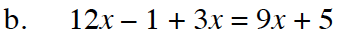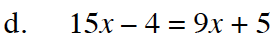Home > CC4 > Chapter 11 > Lesson 11.2.5 > Problem11-106

11-106.
1. Which of the equations below is equivalent to 4(3x − 1) + 3x = 9x + 5? More than one may be equivalent. Justify your answer. Homework Help ✎

2.  a. 12x − 4 + 3x = 9x + 5 b. 12x − 1 + 3x = 9x + 5 c. 11x = 14x d. 15x − 4 = 9x + 5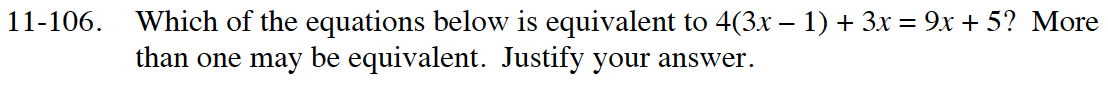Write out the steps for solving the original equation. Which of the equations match one of your steps?